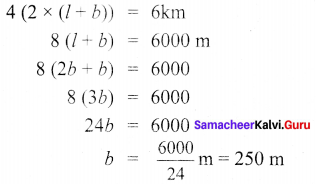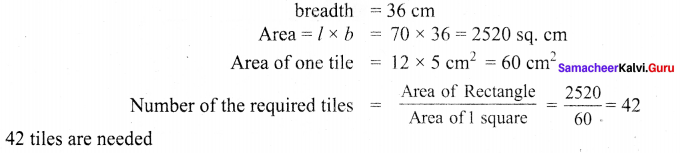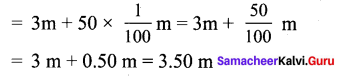# Samacheer Kalvi 6th Maths Solutions Term 3 Chapter 3 Perimeter and Area Additional Questions## Tamilnadu Samacheer Kalvi 6th Maths Solutions Term 3 Chapter 3 Perimeter and Area Additional Questions

Question 1.
The length of a rectangular field is twice its breadth. Ramu runs around it four times and covered a distance of 6 km. What is the length of the field.
Solution:
Breadth of rectangular field = b m
Length of the field = 2b m
Distance covered in one round = perimeter
Distance covered in 4 rounds = 4 × perimeterLength of the field = 2 × 250 = 500 mQuestion 2.
How many tiles whose length and breadth are 12 cm and 5 cm respectively will be needed to fit in a rectangular region whose length and breadth are 70 cm and 36 cm?
Solution:
Given length of the rectangular region = 70 cmQuestion 3.
A room is 4m long and 3m 50cm wide, How many square metres of carpet is needed to cover the floor of the room?
Solution:
Given length of the room = 4 m
Breadth = 3 m 50 cm = 3 m + 50 cmCarpet needed = Area of the floor = length × breadth
= 4 × 3.5 m = 14 sq. mQuestion 4.
Find the area of the square Whose side is 14 cm.
Solution:
Side of the square = 14 cm
Area of the square = side × side = (14 × 14) cm2 = 196 sq cm.

Question 5.
Two sides of a triangle are 12 cm and 14 cm. The perimeter of the triangle is 36 cm. What is its third side.
Solution:
Given two sides of a triangle = 12 cm and 14 cm
∴ Perimeter = 36 cm
12 cm + 14 cm + third side = 36 cm
Third side = 36 – 26 = 10 cm
∴ Length of the third side